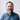# JavaScript Equality

A few weeks ago, I built an app with React.js and create-react-app that I call ‘JavaScript Equality’. It’s deployed here:

https://javascript-equality.now.sh/

This application demonstrates the JavaScript value-comparison operators `==` and `===`. It’s inspired by the JavaScript Equality Table, an app I stumbled across while trying to get a better understanding of JavaScript equality.The image above shows why the ‘threequals’ (`===`) is superior to the double equals (`==`). If you want true equality, the kind of logic you’d expect with any other programming language, use the threequals. It is the predictable choice.

My favorite implementation detail of this app is the data model, which is created in the constructor function:

``````const comparatorArray = this.axis().map(() => this.axis().slice(0));
const dataModel = this.axis().map((xValue, index) =>
comparatorArray[index].map(yValue => ({
// eslint-disable-next-line
twoquals: yValue == xValue,
threequals: yValue === xValue,
}))
);``````

What I do here is create an array of arrays, `comparatorArray`, that has all the comparators (`true`, `false`, etc.) in the axis, in order, with as many indexes as there are comparators (a square). Then I map over the axis and compare each comparator to each value using double and triple equals. This creates a data model like so for a 4 x 4 square of values:

``````[
[
{ twoquals: true, threequals: true },
{ twoquals: false, threequals: false },
],
[
{ twoquals: false, threequals: false },
{ twoquals: true, threequals: true },
],
];``````

From here we can toggle a state value `view` between `twoquals` and `threequals`. All math happens in the initial state.

I’ll close with a quote from Douglas Crawford:

My advice is to never use the evil twins. Instead, always use `===` and `!==`.

Edit on GitHub

Each week, I write an email about React. It's a collection of quotes, news, conference talks, and documentation curated be me to help you get up to speed on this exploding ecosystem. Join my subscribers today by subscribing to React Explained.